# DAV Class 4 Maths Chapter 7 Brain Teasers Solutions

The DAV Class 4 Maths Solutions and DAV Class 4 Maths Chapter 7 Brain Teasers Solutions of Capacity offer comprehensive answers to textbook questions.

## DAV Class 4 Maths Ch 7 Brain Teasers Solutions

Question 1.
(a) The unit to measure the length of a railway track is-
(i) metre
(ii) centimetre
(iii) kilometre
(iv) gram
(iii) kilometre

(b) 3 m 3 cm = __________ cm
(i) 33
(ii) 303
(iii) 330
(iv) 3300
(ii) 303

(c) For a family of three, rice needed for one meal is __________
(i) 4$$\frac{1}{2}$$ kg
(ii) 1500 g
(iii) $$\frac{1}{4}$$ kg
(iv) 1000 g
(iii) $$\frac{1}{4}$$ kg

(d) Which of following is not sold by measuring capacity?
(i) milk
(ii) petrol
(iii) apples
(iv) oil
(iii) apples

(e) The smallest unit of weight is-
(i) milligram
(ii) gram
(iii) centimetre
(iv) millimetre
(i) milligramQuestion 2.
Which unit of measurement will you choose to express the following?
(a) length of a railway track
(b) Weight of an apple
(c) ink in a pen
(d) height of a 10-year old boy.
(a) km
(b) gram
(c) ml
(d) cm, m

Question 3.
Fill in the blanks.
(a) 7 kg = __________g
(b) 10 m = __________cm
(c) 72 l = __________ml
(d) 2 kg 5 g __________g
(e) 3 m 3 cm = __________cm
(f) 9 l 352 ml = __________ ml
(g) 7050 ml = __________l __________ml
(h) 325 cm = __________m __________cm
(i) 1575 g = __________kg __________ g
(j) 7 km = __________m
(a) 7000
(b) 1000
(c) 72000
(d) 2005
(e) 303
(f) 9352
(g) 7 l 50 ml
(h) 3 m 25 cm
(i) 1 kg 575 g
(j) 7000

Question 4.
Find the sum of:
(a) 3 kg 520 g, 7 kg 95 g and 11 kg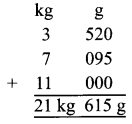21 kg 615 g

(b) 11m 70 cm, 9 m 95 cm and 16 m 2 cm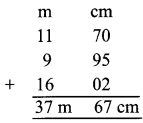37 m 67 cm

Question 5.
Find the difference of:
(a) 49 kg and 31 kg 286 g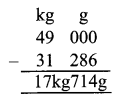17kg 714 g

(b) 13l 479 ml and 9l 293 ml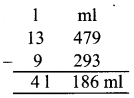4l 186 ml

Question 6.
A basket contained 65 kg 750 g of fruits. Out of which 42 kg 150 g are apples, 9 kg 750 g are pears and rest are mangoes. Find the weight of mangoes.
To get the wt. of mangoes, we have to subtract the wt. of apples and pears from total wt.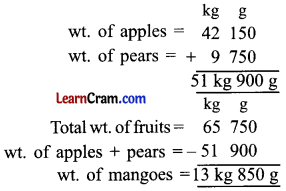13 kg 850 g of mangoes.

Question 7.
A tall tower is painted in red, white and black colour, 25 m 50 cm is painted black, 15 m 75 cm is painted red and 10 m 25 cm is painted white. Find the height of the tower.
To get total height we have to add the length of colours. m cm
Length of black colour = 25m 50cm
Length of red colour = 15m 75cm
Length of white colour = 10m 25cm
Height of tower = 51 m 50 cm51 m 50 cm is the height of tower.Question 8.
For a family of three, how much of each given item would you buy for one meal? Circle your answer.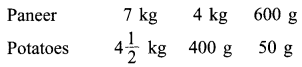Paneer – 600 g
Potatoes – 400 g

Question 1.
Convert the following into millilitres.
(a) 19 l 10 ml
19 1 10 ml
= 19 × 1000 ml + 10 ml
= 19010 ml

(b) 90 l
= 90 × 1000
= 90,000 ml

(c) 101 l 37 ml
= 101 × 1000ml + 37 ml
= 101037 ml

Question 2.
Convert the following into litres.
(a) 177800 ml
(b) 7580 ml
(c) 90003 ml
(a) 177800 ml
= 177800 – 1000 l
= 177 l 800 ml

(b) 7580 ml
= 7580 – 1000 l
= 7 1 580 ml

(c) 90003 ml
= 90003 ÷ 1000
= 90 1 3 ml

Question 3.
(a) 126 1 26 ml and 39 1 36 ml165l 062 ml

(b) 60 1 7 ml and 80 1 230 ml140l 237 ml

(c) 76 1 152 ml, 36 1 56 ml and 50 1 36 ml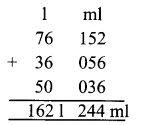162l 244 ml

Question 4.
Subtract:
(a) 36 l 56 ml and 96 l 78 ml60l 022 ml

(b) 156 l 840 ml and 130 l 970 ml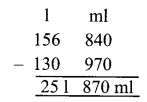25l 870 ml

(c) 15 l 480 ml and 96 l 20 ml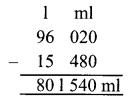80 l 540 ml.Question 5.
A shopkeeper ordered for 90 l 70 ml of milk. But he received only 87 1 26 ml of milk. Due to leakage in packet of milk, some milk got wasted. Find the amount of milk got wasted.
To get wasted milk we have to subtract
Quantity of milk ordered = 90l 070ml
Quantity of milk he received = 87l 026ml
Milk got wasted = 3l 044 ml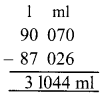3 l 44 ml of milk.

Question 6.
Fill in the blanks.
(a) 72 l 36 ml = __________ ml